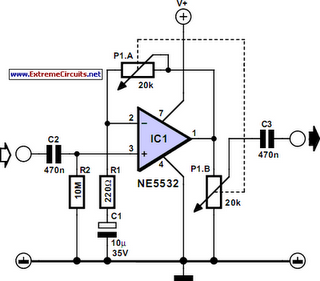# Learning Electronics

Learn to build electronic circuits

# Zero Gain Mod For Non-Inverting Opamp

Electronics textbooks will tell you that a non-inverting opamp normally cannot be regulated down to 0 dB gain. If zero output is needed then it is usual to employ an inverting amplifier and a buffer amp in front of it, the buffer acting as an impedance step-up device. The circuit shown here is a trick to make a non-inverting amplifier go down all the way to zero output. The secret is a linear-law stereo potentiometer connected such that when the spindle is turned clockwise the resistance in P1a increases (gain goes up), while the wiper of P1b moves towards the opamp output (more signal). When the wiper is turned anti-clockwise, the resistance of P1a drops, lowering the gain, while P1b also supplies a smaller signal to the load. In this way, the output signal can be made to go down to zero.

Circuit diagram:Zero Gain Mod Circuit Diagram For Non-Inverting Opamp